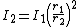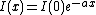# attenuation

(redirected from Attentuate)
Also found in: Dictionary, Thesaurus, Medical.
Related to Attentuate: attenuate

## Attenuation

The reduction in level of a transmitted quantity as a function of a parameter, usually distance. It is applied mainly to acoustic or electromagnetic waves and is expressed as the ratio of power densities. Various mechanisms can give rise to attenuation. Among the most important are geometrical attenuation, absorption, and scattering.

For unconfined radiation from a point source in free space, the power density (watts per square meter) decreases in proportion to the square of the distance. The power densities, I1 and I2, at distances r1 and r2 from the source, are related by Eq. (1).

(1)If the signal, in a parallel beam so that there is no geometrical attenuation, passes through a lossy medium, absorption reduces the power level, I, exponentially with distance, x, according to Eq. (2), where a

(2)is the attenuation coefficient. See Absorption of electromagnetic radiation, Sound absorption

Scattering is said to occur if the power is not absorbed in the medium but scattered from inhomogeneities. See Scattering of electromagnetic radiation

More complicated situations occur with guided waves, such as acoustic waves in pipes or electromagnetic waves in transmission lines or waveguides, where absorption may take place and irregularities may cause reflection of some power.

In electric circuits, constituent elements are often described as attenuators when they reduce the level of signals passing through them.

Attenuation is usually measured in terms of the logarithm of the power ratio, the units being the neper or the decibel. See Decibel

## attenuation

(ă-ten-yoo-ay -shŏn) The reduction in magnitude of a quantity as a function of distance from source or of some other parameter. The attenuation of a beam of radiation, i.e. the reduction in intensity or flux density, results from absorption and/or scattering of the waves or particles as they pass through matter.

## attenuation

[ə‚ten·yə′wā·shən]
(botany)
Tapering, sometimes to a long point.
(electricity)
The exponential decrease with distance in the amplitude of an electrical signal traveling along a very long uniform transmission line, due to conductor and dielectric losses.
(engineering)
A process by which a material is fabricated into a thin, slender configuration, such as forming a fiber from molten glass.
(microbiology)
Weakening or reduction of the virulence of a microorganism.
(physics)
The reduction in level of a quantity, such as the intensity of a wave, over an interval of a variable, such as the distance from a source.

## sound attenuation

The reduction in the intensity or in the sound pressure level of sound which is transmitted from one point to another. Also see sound insulation.

## attenuation

The decrease in the intensity of a signal, beam, or wave as a result of the absorption of energy (by the terrain or medium over which, or through which, the energy travels) and of scattering out of the path to the detector but not including the reduction as a result of geometric spreading. Attenuation is usually expressed in dB (decibles). The term is often used as a misnomer for attenuation coefficient, which is expressed in dB per kilometer.

## attenuation

(communications)
The progressive reduction in amplitude of a signal as it travels farther from the point of origin.

For example, an electric signal's amplitude reduces with distance due to electrical impedance. Attenuation is usually measured in decibels Attenuation does not imply appreciable modification of the shape of the waveform (distortion), though as the signal amplitude falls the signal-to-noise ratio will also fall unless the channel itself is noise free or the signal is amplified at some intermediate point(s) along the channel.

["Networking Essentials, second edition", Microsoft Corporation, pub. Microsoft Press 1997].

## attenuation

Loss of signal power in a transmission. Often abbreviated "ATTN."
Site: Follow: Share:
Open / Close